# Electric Current Lesson 9 Electric Current Electric current

• Slides: 15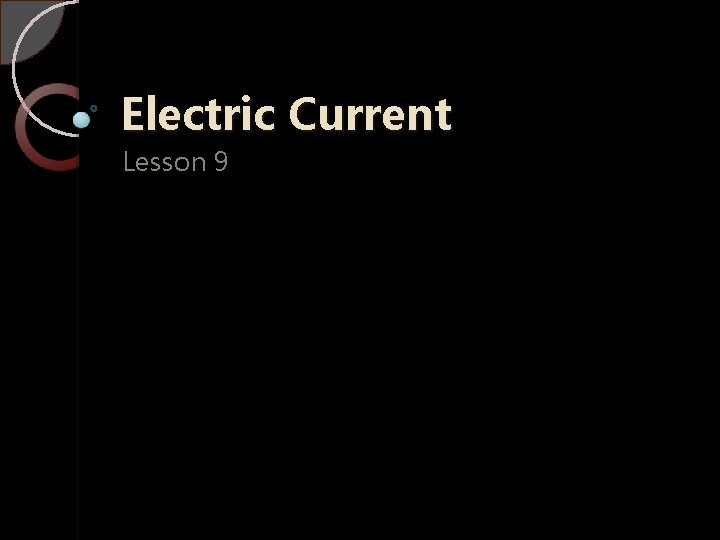Electric Current Lesson 9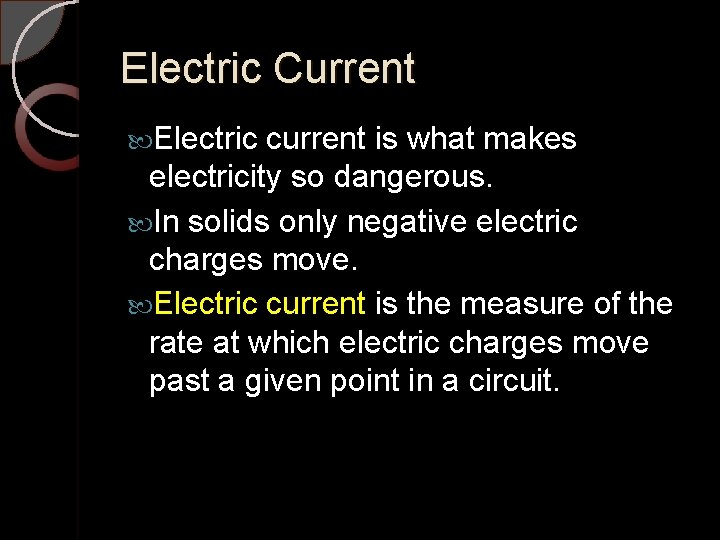Electric Current Electric current is what makes electricity so dangerous. In solids only negative electric charges move. Electric current is the measure of the rate at which electric charges move past a given point in a circuit.Electric Current The metric SI unit used to measure electric current is the ampere. The symbol for ampere is A. Slightly less than one ampere (1 A) flows through a 100 W light bulb in a 120 V circuit. Current is measured by using an ammeter connected to the circuit in series.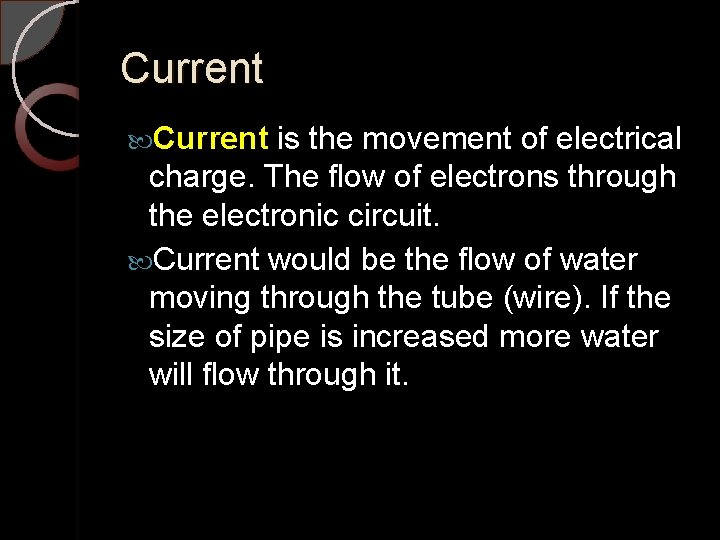Current is the movement of electrical charge. The flow of electrons through the electronic circuit. Current would be the flow of water moving through the tube (wire). If the size of pipe is increased more water will flow through it.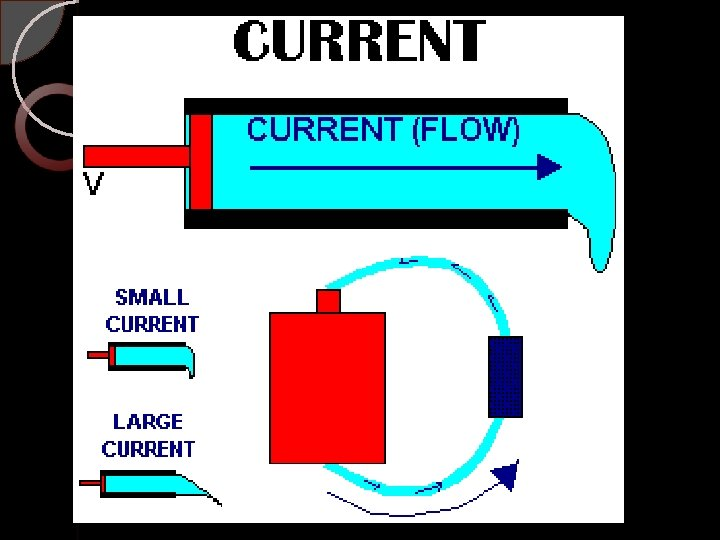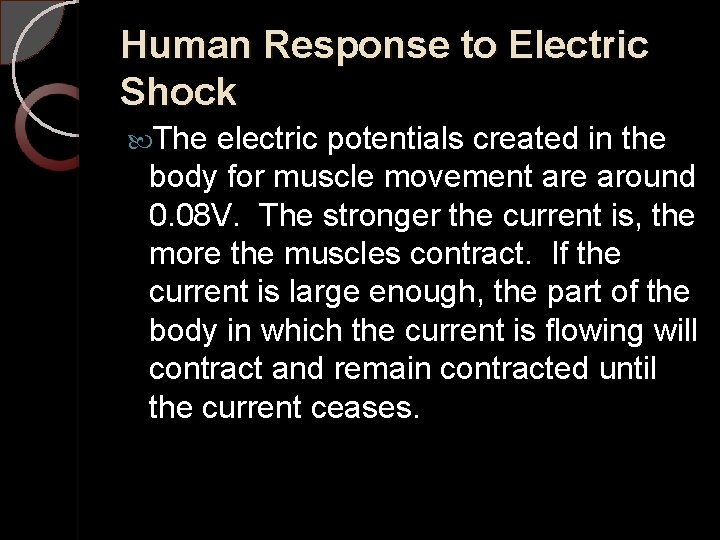Human Response to Electric Shock The electric potentials created in the body for muscle movement are around 0. 08 V. The stronger the current is, the more the muscles contract. If the current is large enough, the part of the body in which the current is flowing will contract and remain contracted until the current ceases.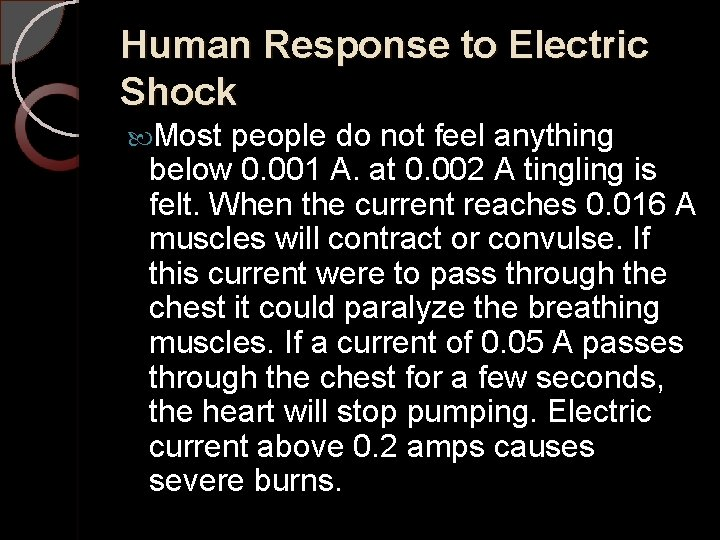Human Response to Electric Shock Most people do not feel anything below 0. 001 A. at 0. 002 A tingling is felt. When the current reaches 0. 016 A muscles will contract or convulse. If this current were to pass through the chest it could paralyze the breathing muscles. If a current of 0. 05 A passes through the chest for a few seconds, the heart will stop pumping. Electric current above 0. 2 amps causes severe burns.Electrical ResistanceElectrical Resistance All types of conductors impede the flow of electrons to some extent. This is called electrical resistance. Some kinds of electrical devices are designed for this purpose and are called resistors.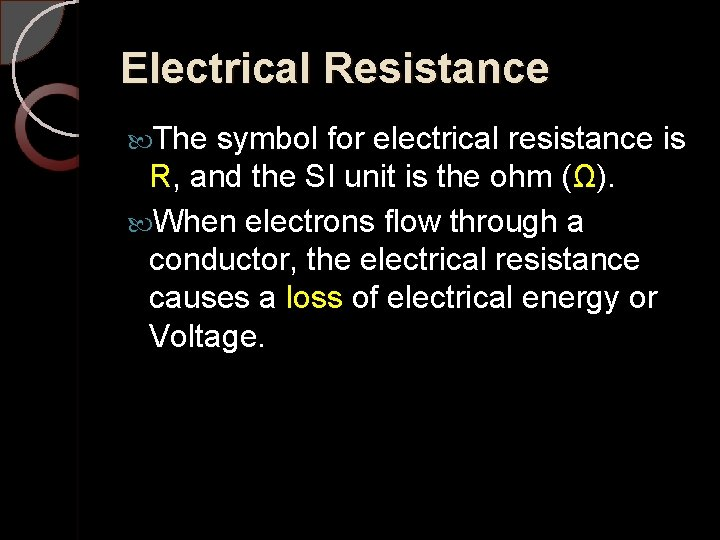Electrical Resistance The symbol for electrical resistance is R, and the SI unit is the ohm (Ω). When electrons flow through a conductor, the electrical resistance causes a loss of electrical energy or Voltage.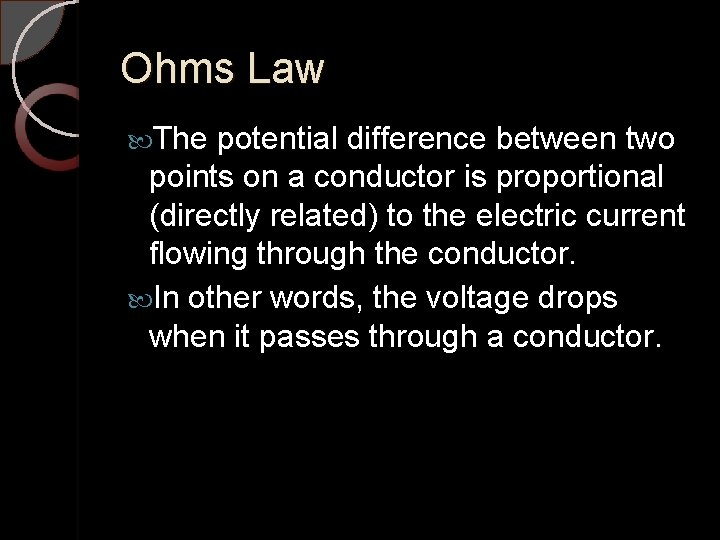Ohms Law The potential difference between two points on a conductor is proportional (directly related) to the electric current flowing through the conductor. In other words, the voltage drops when it passes through a conductor.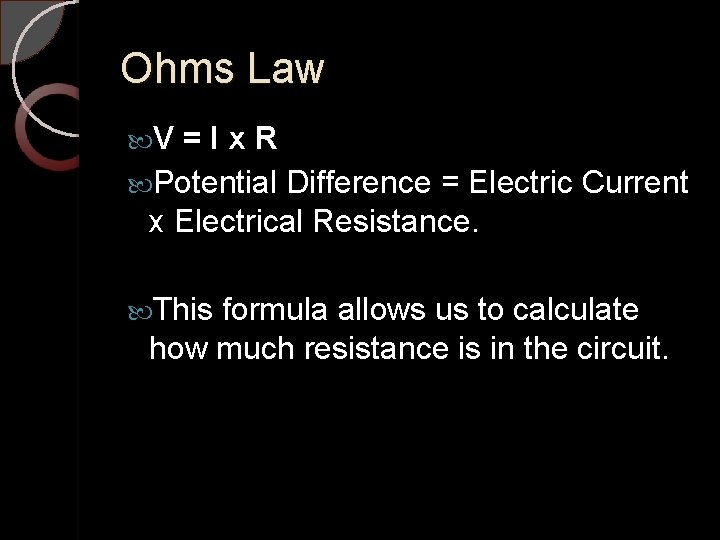Ohms Law V =Ix. R Potential Difference = Electric Current x Electrical Resistance. This formula allows us to calculate how much resistance is in the circuit.Example What is the voltage of a car battery with 800 Amps of current and 0. 015 Ohms? I = 800 A R = 0. 015 Ω V = ?V =Ix. R V = (800 A) x (0. 015 Ω) = 12 V Therefore, the car battery is 12 V.Measuring Current in a Circuit Lab